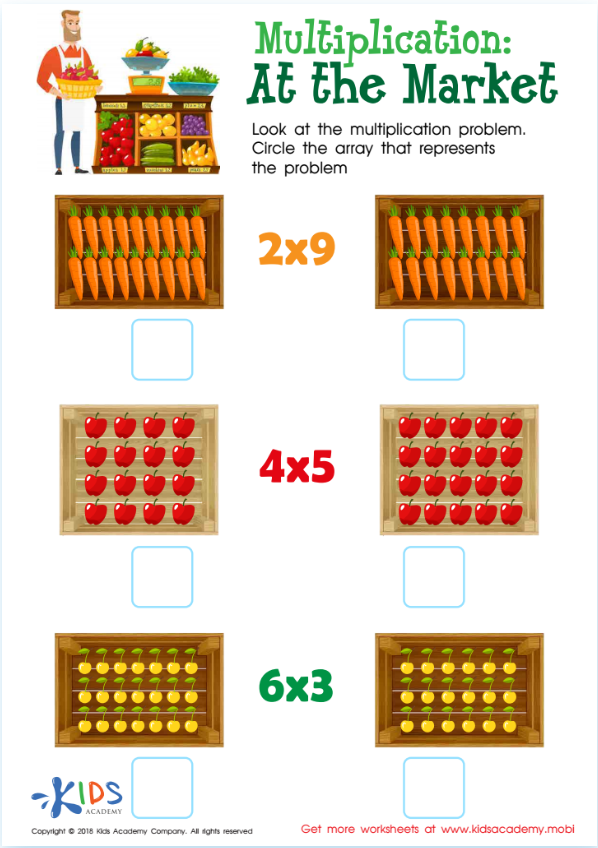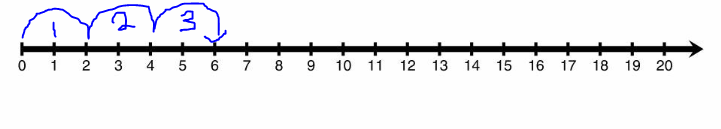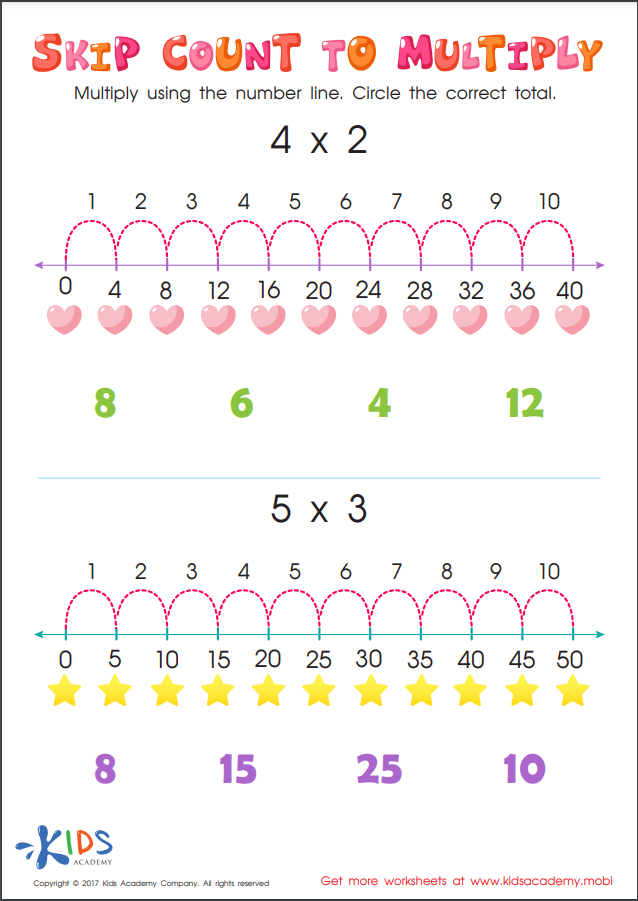All Posts

# Using Arrays and Skip Counting to Teach Multiplication

April 26, 2022

If your child is having some trouble mastering more advanced math problems like division or two-digit multiplication, it might be worth revisiting their basic multiplication facts. Practicing multiplication facts doesn’t have to involve tedious drills and boring flash cards though! In fact, it is most important that your child understands the concept of multiplication first. In this article, you’ll learn how visuals such as arrays and skip counting on a number line can help your child visualize how multiplication works.

Kids will often confuse multiplication for addition or memorize various multiplication facts without actually understanding what multiplication is. So what does it mean to multiply? When we multiply, we are actually adding equal groups. For example, the problem 2 “times” 3 means that we are adding a group of 2, three times. Multiplication has what we call a commutative property. This characteristic of multiplication means that no matter what order we multiply the numbers in, the answer is the same. This means that we might think of “2 times 3” as adding a group of 2, three times OR we might think of it as adding a group of 3, two times.

One of the easiest ways for your child to visualize this is with an array. You might think of an array as an evenly distributed arrangement of objects, such as numbers, in rows and columns, similar to a grid. A rectangular array can be used to help your child visualize and understand multiplication in a more concrete way. If you need more information about what an array is, check out this video called, “Arrays for Kids.”Another technique used to visualize multiplying iis skip counting using a number line. You might start by drawing a basic number line that is numbered from zero to ten. Then, explain that a multiplication problem like 2x3 is actually asking you what you get if you make three groups of 2. Notice how in the number line below, creating 2 groups of 3 shows why six is the product of 2x3.Encourage your child to practice multiplying using number lines with this Skip Count Multiplication activity. In this activity, the number lines show skip counting by 4s and 5s. Work with your child to skip count the assigned amount of times in order to “land” on the correct answer on the number line.For more worksheets and games on the topic above, install the Talented and Gifted app.

Mobile version
SHARE